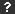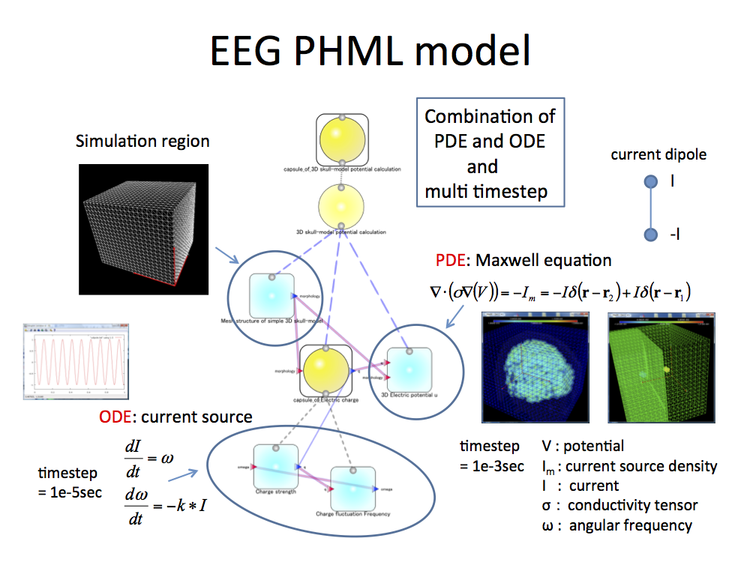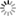##### SectionsChoose below to refine your search
Research Topic

Download the NI2012 abstract book here. The page numbers in the index are clickable for easy browsing.

# EEG Analysis in PhysioDesigner

Filed under:
1.57894736842

Hideki Oka (NIJC, RIKEN BSI), Ken-ichiro Iwasaki (NIJC,RIKEN BSI), Yoshiyuki Asai (OIST), Taishin Nomura (Department of Mechanical Science and Bioengineering, Osaka University), Yoko Yamaguchi (NIJC, RIKEN BSI)

PhysioDesigner is Integrated Development Environment for physiome biological modeling based on modeling description language PHML. It can handle ODE, DDE, SDE and PDE. Here we will show how EEG (electroencephalogram) analysis can be done under this environment. EEG analysis PHML model is shown in Fig. 1. EEG model is composed of three parts, morphology module, Maxwell equation module and current source capsule module. Current source capsule module is composed of current strength module and frequency module. Morphology module gives cubic simulation region information in which brain structure is stored. Current source module gives information of rhythmic current source which is an origin of EEG signal. Maxwell equation module solves Maxwell equation which needs conductivity values extracted from brain image and gives EEG signal. Brain conductivity is extracted from CT image of grey, white, csf and skull images. For the purpose, image processing tool “Imageviewer” has been developed. Imageviewer has four functions, image, extract, smoothing and resolution. Conductivity matrix file is generated from Imageviewer and is used for Maxwell equation to get electric potentials.
To solve partial differential equation, finite element method is used because of its adaptability to complex structure and high numerical accuracy. We adopt a script based solver FreeFem++. Conductivity file is read from FreeFem++ and its values are assigned to each cubic point. Current source is a dipole whose current strength rhythmically vibrates at a decided frequency. Current strength q and angular frequency ω obey following two equations. In this case, αwave of 10Hz is assumed. EEG PHML model has two kinds of equations, Maxwell PDE and current source ODE. Each transient equation runs at different time step. In PhysioDesigner, FreeFem++ parser reads multi time step information and assigns it to each equation to run it at different time step. EEG signal is observed at standard configuration electrodes. In this case, 64 channel electrodes is adopted and MNI standard coordinates conversion is done automatically. This configuration is stored in one segment of cuboid.  http://physiodesigner.org  http://www.frefem.orgIllustration of EEG PHML model
Preferred presentation format: Poster
Topic: Computational neuroscience

Filed under: